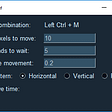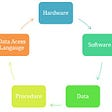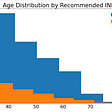# Operational Research

For most people, when they first time encountered “Operational Research”, they do not know what it means. Research on how to operate staff? To give simple explanation, it is research for “optimisation”, in other words, science for “better”.

First, we will introduce 3 basic types of Optimisation. Then we will classify real life problems into different tasks natures.

We all know there are existing tools or algorithms to solve each generic types of problems. The KEY is could we “transform” real life problems to one of the generic types, then utilise the tools to solve them.

Linear Optimisation

If a problem can be modelled as a set of linear relationships, then it can be solved by linear optimisation. Usually problem modelling involves 3 parts, variables, constraints and objective.

`# variables: x, y# Objective: maximize 3x + 2y# Constraints:x >= 0y >= 32x + y < 100`

Constraint Optimisation

Constraint optimisation does not necessarily have an objective compared to linear optimisation. Therefore, it is normal that optimal solution could not be found. Usually there will be a lot of feasible solutions. as long as the constraints all got meet up.

Integer Optimisation

Some variables might need to be integers only, it is very common in real life situation. It is also called MIP(Mixed Integer Programming).

We will describe several generic tasks Operational Research will be good at solving. If you can transform a problem to any one of these types, very likely you can easily find an answer.

Above chart listed out most generic types of operational research, KEY of solving problems is to transform real life situation to one of these types. Then you can make use of the tools or algorithms to solve the problems easily. Modelling a real life situation to a mathematical case is a very important ability.

There are several commercial software you can use for OR. But here we introduce Google’s open source library OR-Tools. Once you can model your real life problem into operational situation, you can use tools like this one to find the answers.

A simple linear optimisation in Python will look like this:

`from ortools.linear_solver import pywraplpsolver = pywraplp.Solver('test', pywraplp.Solver.GLOP_LINEAR_PROGRAMMING)# variables, setting boundaryx = solver.NumVar(0, 100, 'x')y = solver.NumVar(0, 100, 'y')z = solver.NumVar(0, 100, 'z')solver.NumVariables()# constraintsct = solver.Constraint(0, 200, 'ct')ct.SetCoefficient(x, 1)ct.SetCoefficient(y, 1)solver.NumConstraints()# objectiveobjective = solver.Objective()objective.SetCoefficient(x, 1)objective.SetCoefficient(y, 2)objective.SetCoefficient(z, -3)objective.SetMaximization()print(f"number of variables: {solver.NumVariables()}")print(f"number of constraints: {solver.NumConstraints()}")status = solver.Solve()print(f"solved value: {objective.Value()}")print("x, y, z values:",x.solution_value(),y.solution_value(),z.solution_value())`

--

--

--

## More from datalearningpr

Love podcasts or audiobooks? Learn on the go with our new app.

## How To Install Odoo x Docker on Ubuntu 16.04## Taking the Associate Android Developer Exam## Automice — Mouse auto-mover## A Text Analysis of Women Clothing Reviews## [Tech Update] Horizon Exchange Progress## Managing the privacy of your Kerika boards## What’s so fuzzy about our reasoning?## What skills should I add to my arsenal?## Statistical Analysis for an Insurance Firm in Singapore (Part 5 of 7)